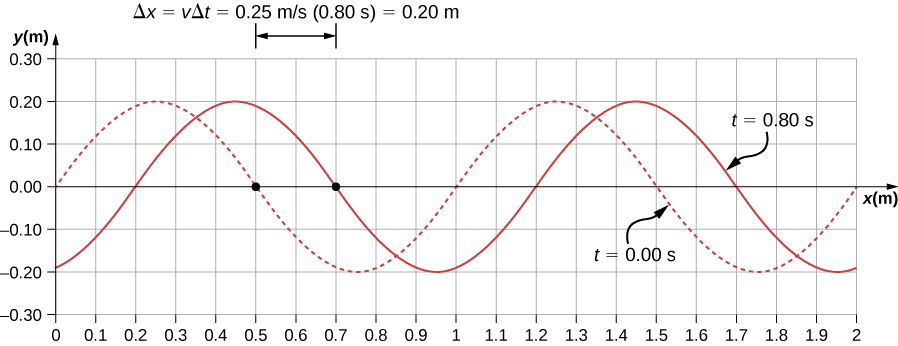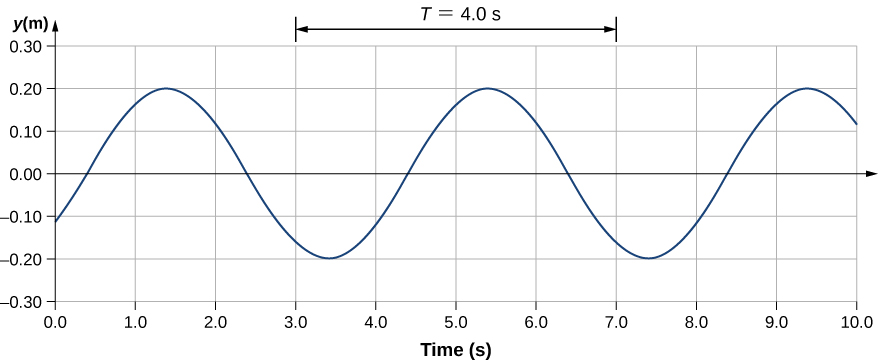# 16.2 Mathematics of waves  (Page 3/11)

 Page 3 / 11

## Characteristics of a traveling wave on a string

A transverse wave on a taut string is modeled with the wave function

$y\left(x,t\right)=A\phantom{\rule{0.2em}{0ex}}\text{sin}\left(kx-wt\right)=0.2\phantom{\rule{0.2em}{0ex}}\text{m}\phantom{\rule{0.2em}{0ex}}\text{sin}\left(6.28\phantom{\rule{0.2em}{0ex}}{\text{m}}^{-1}x-1.57\phantom{\rule{0.2em}{0ex}}{\text{s}}^{-1}t\right).$

Find the amplitude, wavelength, period, and speed of the wave.

## Strategy

All these characteristics of the wave can be found from the constants included in the equation or from simple combinations of these constants.

## Solution

1. The amplitude, wave number, and angular frequency can be read directly from the wave equation:
$y\left(x,t\right)=A\phantom{\rule{0.2em}{0ex}}\text{sin}\left(kx-wt\right)=0.2\phantom{\rule{0.2em}{0ex}}\text{m}\phantom{\rule{0.2em}{0ex}}\text{sin}\left(6.28\phantom{\rule{0.2em}{0ex}}{\text{m}}^{-1}x-1.57\phantom{\rule{0.2em}{0ex}}{\text{s}}^{-1}t\right).$
$\left(A=0.2\phantom{\rule{0.2em}{0ex}}\text{m;}\phantom{\rule{0.2em}{0ex}}k=6.28\phantom{\rule{0.2em}{0ex}}{\text{m}}^{-1};\phantom{\rule{0.2em}{0ex}}\omega =1.57\phantom{\rule{0.2em}{0ex}}{\text{s}}^{-1}\right)$
2. The wave number can be used to find the wavelength:
$\begin{array}{}\\ k=\frac{2\pi }{\lambda }.\hfill \\ \lambda =\frac{2\pi }{k}=\frac{2\pi }{6.28\phantom{\rule{0.2em}{0ex}}{\text{m}}^{-1}}=1.0\phantom{\rule{0.2em}{0ex}}\text{m}.\hfill \end{array}$
3. The period of the wave can be found using the angular frequency:
$\begin{array}{}\\ \\ \omega =\frac{2\pi }{T}.\hfill \\ T=\frac{2\pi }{\omega }=\frac{2\pi }{1.57\phantom{\rule{0.2em}{0ex}}{\text{s}}^{-1}}=4\phantom{\rule{0.2em}{0ex}}\text{s}.\hfill \end{array}$
4. The speed of the wave can be found using the wave number and the angular frequency. The direction of the wave can be determined by considering the sign of $kx\mp \omega t$ : A negative sign suggests that the wave is moving in the positive x -direction:
$|v|=\frac{\omega }{k}=\frac{1.57\phantom{\rule{0.2em}{0ex}}{\text{s}}^{-1}}{6.28\phantom{\rule{0.2em}{0ex}}{\text{m}}^{-1}}=0.25\phantom{\rule{0.2em}{0ex}}\text{m/s}.$

## Significance

All of the characteristics of the wave are contained in the wave function. Note that the wave speed is the speed of the wave in the direction parallel to the motion of the wave. Plotting the height of the medium y versus the position x for two times $t=0.00\phantom{\rule{0.2em}{0ex}}\text{s}$ and $t=0.80\phantom{\rule{0.2em}{0ex}}\text{s}$ can provide a graphical visualization of the wave ( [link] ).A graph of height of the wave y as a function of position x for snapshots of the wave at two times. The dotted line represents the wave at time t = 0.00 s and the solid line represents the wave at t = 0.80 s . Since the wave velocity is constant, the distance the wave travels is the wave velocity times the time interval. The black dots indicate the points used to measure the displacement of the wave. The medium moves up and down, whereas the wave moves to the right.

There is a second velocity to the motion. In this example, the wave is transverse, moving horizontally as the medium oscillates up and down perpendicular to the direction of motion. The graph in [link] shows the motion of the medium at point $x=0.60\phantom{\rule{0.2em}{0ex}}\text{m}$ as a function of time. Notice that the medium of the wave oscillates up and down between $y=+0.20\phantom{\rule{0.2em}{0ex}}\text{m}$ and $y=-0.20\phantom{\rule{0.2em}{0ex}}\text{m}$ every period of 4.0 seconds.A graph of height of the wave y as a function of time t for the position x = 0.6 m . The medium oscillates between y = + 0.20 m and y = −0.20 m every period. The period represented picks two convenient points in the oscillations to measure the period. The period can be measured between any two adjacent points with the same amplitude and the same velocity, ( ∂ y / ∂ t ) . The velocity can be found by looking at the slope tangent to the point on a y -versus- t plot. Notice that at times t = 3.00 s and t = 7.00 s , the heights and the velocities are the same and the period of the oscillation is 4.00 s.

Check Your Understanding The wave function above is derived using a sine function. Can a cosine function be used instead?

Yes, a cosine function is equal to a sine function with a phase shift, and either function can be used in a wave function. Which function is more convenient to use depends on the initial conditions. In [link] , the wave has an initial height of $\text{y}\left(0.00,0.00\right)=0$ and then the wave height increases to the maximum height at the crest. If the initial height at the initial time was equal to the amplitude of the wave $\text{y}\left(0.00,0.00\right)=\text{+}A,$ then it might be more convenient to model the wave with a cosine function.

a length of copper wire was measured to be 50m with an uncertainty of 1cm, the thickness of the wire was measured to be 1mm with an uncertainty of 0.01mm, using a micrometer screw gauge, calculate the of copper wire used
If centripetal force is directed towards the center,why do you feel that you're thrown away from the center as a car goes around a curve? Explain
Which kind of wave does wind form
calculate the distance you will travel if you mantain an average speed of 10N m/s for 40 second
hw to calculate the momentum of the 2000.0 elephant change hunter at a speed of 7.50 m/s
how many cm makes 1 inches
2.5
omwoyo
2.54cm=1inche
omwoyo
how do we convert from m/s to km/hr
When paddling a canoe upstream, it is wisest to travel as near to the shore as possible. When canoeing downstream, it may be best to stay near the middle. Explain why?
Explain why polarization does not occur in sound
one ship sailing east with a speed of 7.5m/s passes a certain point at 8am and a second ship sailing north at the same speed passed the same point at 9.30am at what distance are they closet together and what is the distance between them then
density of a subtance is given as 360g/cm,put it in it s.i unit form
if m2 is twice of m1. find the ration of kinetic energy in COM system to lab system of elastic collision
What is a volt equal to?
list and explain the 3 ways of charging a conductor
conduction convention rubbing
Asdesaw
formula of magnetic field
why polarization does not occur in sound
Integral of a vector
define surface integral of a vector?
Rahat

#### Get Jobilize Job Search Mobile App in your pocket Now!By OpenStaxBy Edgar DelgadoBy OpenStaxBy OpenStaxBy Mary CohenBy OpenStaxBy John GabrieliBy Wey HeyBy Nicole DuquetteBy Gerr Zen